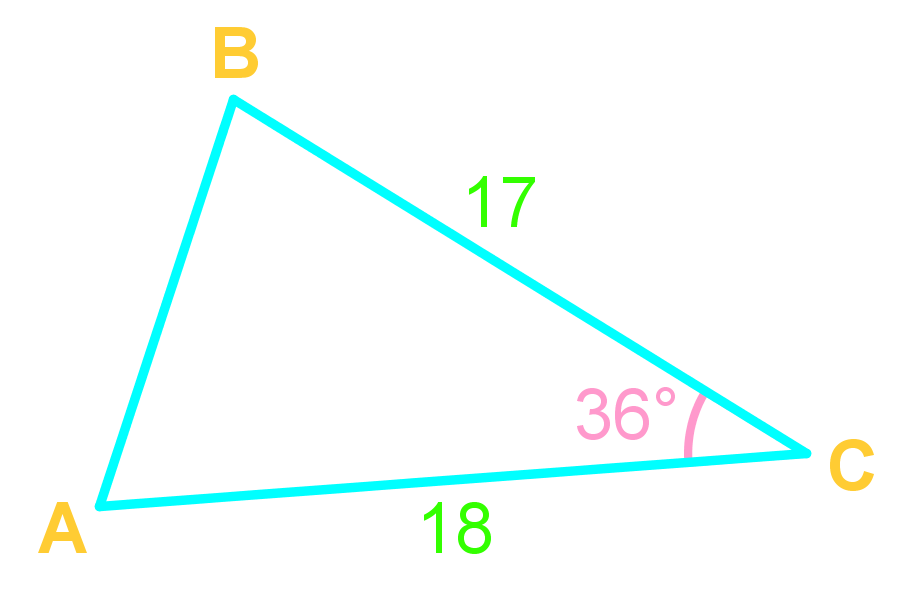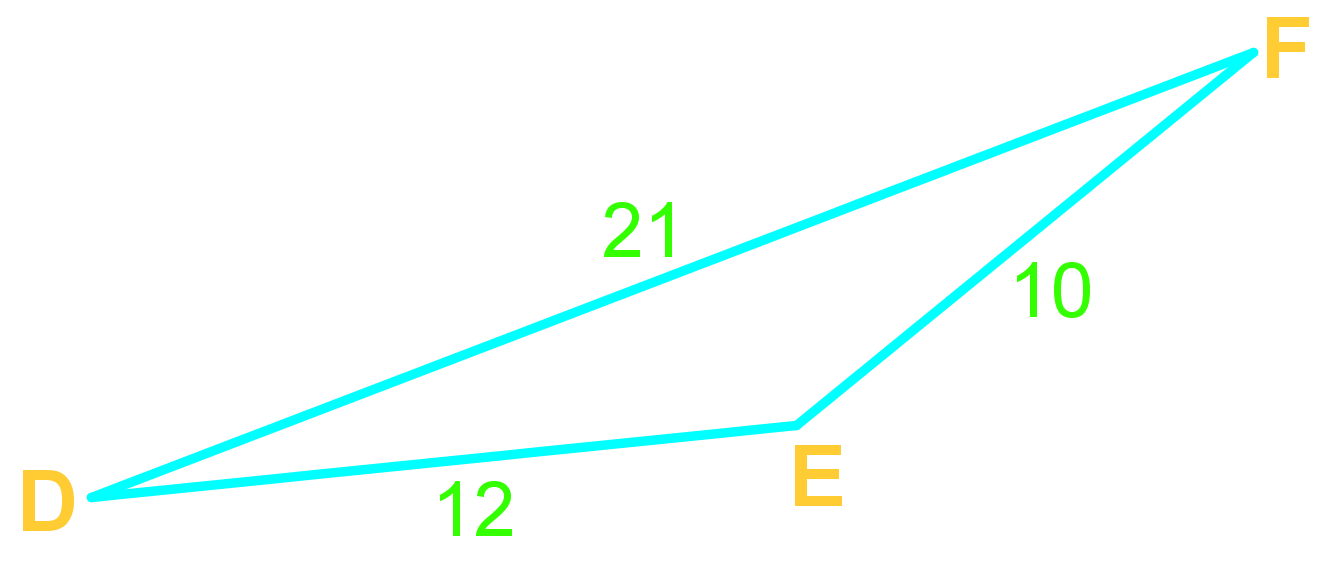# Law of cosines

### Law of cosines

There are times when the Law of Sines cannot solve the triangle because we encounter a triangle with either one of the following two scenarios – SAS (Side-angle-side), or SSS (Side-Side-Side). What should we do then? We consider using the Law of Cosines, which is another formula that models the relationship between the sides and the angles of any triangle. In this section, we will learn about the concept and the usage of the Law of Cosines, also known as the Cosines Rule.

#### Lessons

For any $\triangle \;ABC$,
$c^2=a^2+b^2-2ab\cos(C)$
Use the Law of Cosine when given SSS (all sides), or SAS (angle-sandwich!)
• Introduction
• What is the law of cosines?
• When and how do we use it?

• 1.
Find the length of side AB• 2.
Find angle E